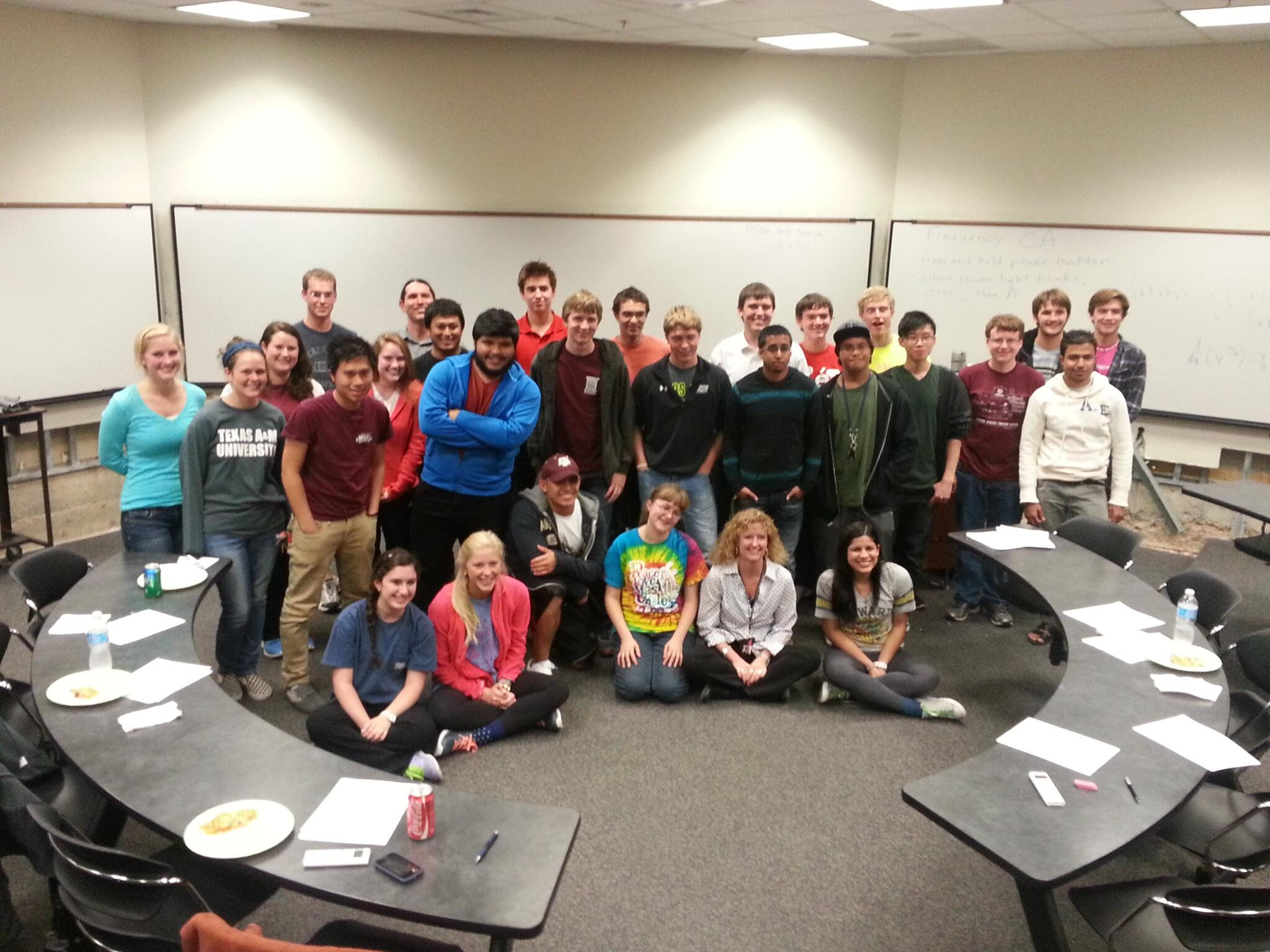# Derivative Bee

Tuesday night was the Math Department’s second annual Derivative Bee, as well as my first visit to the event as a faculty volunteer.Students participate in two categories. Category U is for students currently taking differential calculus (Math 131, 151 and 171). Category G is for students who have completed differential calculus (Math 152, 172 or higher).

In the first round, students are issued clickers and have 3-5 minutes to choose the correct multiple choice answer to a differentiation question. There wasn’t a lot for the faculty volunteers to do at this point, so I thought I’d play along. I was confident of my advantage; this isn’t the first time I’ve taught calculus. And I have those three magic little letters (Ph.D.) following my name.

It didn’t take long for me to get a little attitude adjustment. Question 1 was to evaluate$\dfrac{d}{dx}\left[\dfrac{(x+2)(2x+3)}{x}\right]$

Since I don’t like using the quotient rule, I changed it to a product$\frac{d}{dx}\left[(x+2)(2x+3)x^{-1}\right]$

and promptly made a distribution mistake in the multiple iterations of the product rule required to evaluate the derivative.

What I should have done in the first place was to FOIL out the product and divide through by the x in the denominator$\frac{d}{dx}\left[\dfrac{2x^2 + 7x + 6}{x}\right] = \frac{d}{dx} \left[2x + 7 + \frac{6}{x}\right] = 2 - \frac{6}{x^2}$

which is just oodles easier.

Another problem I got schooled on: find the derivative of$\frac{d}{dx}\sqrt{x\sqrt{x\sqrt{x}}}$

Me? I love the chain rule, and the first thing I did was apply it incorrectly, not realizing that all those embedded square roots were also multiplied together and that the product rule is required, too! Fortunately, I caught my mistake and corrected it — with a not insubstantial amount of sweat. I was all proud of myself until one of the other instructors showed us the easy way to do the problem usingthe rules of exponentials.$\frac{d}{dx}\sqrt{x\sqrt{x\sqrt{x}}} = \frac{d}{dx}(x(x(x)^{\tiny 1/2})^{\tiny 1/2})^{\tiny1/2} = \frac{d}{dx} (x^{\tiny 1/2}x^{\tiny 1/4}x^{\tiny 1/8}) = \frac{d}{dx} (x^{\tiny7/8}) = \frac{7}{8}x^{\tiny -1/8}$

which is an easy problem to solve!

This old dog learned a few tricks tonight. Including renewed respect for my colleagues and students. We have some smart people here at Texas A&M.